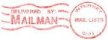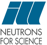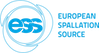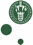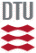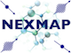McStas: Pol_Bfield Component

# The `Pol_Bfield` Component

Magnetic field component.

## Identification

• Author: Erik B Knudsen, Peter Christiansen and Peter Willendrup
• Origin: RISOE
• Date: July 2011
• Version:(Unknown)

## Description

```
Region with a definable magnetic field.

The component is nestable. This means that it requires a

// START MAGNETIC FIELD
COMPONENT msf =
Pol_Bfield(xwidth=0.08, yheight=0.08, zdepth=0.2, Bx=0, By=-0.678332e-4, Bz=0)
AT (0, 0, 0) RELATIVE armMSF

// HERE CAN BE OTHER COMPONENTS INSIDE THE MAGNETIC FIELD

// STOP MAGNETIC FIELD
COMPONENT msf_stop = Pol_simpleBfield_stop(magnet_comp_stop=msf)
AT (0, 0, 0) RELATIVE armMSF

In between the two component instances the propagation routine
PROP_DT also handles spin propagation.
The current algorithm used for spin propagation is:
SimpleNumMagnetPrecession
in pol-lib.

Example: Pol_Bfield(xwidth=0.1, yheight=0.1, zdepth=0.2, Bx=0, By=1, Bz=0)
Pol_Bfield(xwidth=0.1, yheight=0.1, zdepth=0.2,
filename="bfield.dat", fieldFunction=table_magnetic_field)

The magetic field may be given as any definable c-function with a specific footprint:
int func(double x, double y, double z, double t, double *bx, double *by, double *bz, void *data)
where the magnetic field at coordinates (x,y,z, t) is returned in the variables
pointed to by bx,by, and bz. Additional parameters to be handed to the function
should be handed to the function using the pointer data.

Functions supplied by the system are (the number is the ID of the function to be given as the fieldFunction parameter:
1. Constant magnetic field: Constant field (Bx,By,Bz) within the region
2. Rotating magnetic_field: Field is initially (0,By,0) but after a length of zdepth
has rotated to (By,0,0)
3. majorana magnetic_field: Field is initially (Bx,By,0) liearly transforms to
(-Bx,By,0) after zdepth where By<

Input parameters
Parameters in boldface are required;
the others are optional.

Name  Unit  Description Default
field_type

0
xwidth
m
Width of opening.

0
yheight
m
Height of opening.

0
zdepth
m
Length of field.

0
radius
m
Radius of field if it is cylindrical or spherical.

0
Bx
T
Parameter used for x composant of field.

0
By
T
Parameter used for y composant of field.

0
Bz
T
Parameter used for z composant of field.

0
filename

File where the magnetic field is tabulated.

"bfield.dat"
geometry

NULL
concentric

Allow components and/or other fields inside field. Requires a subsequent Pol_simpleBfield_stop component.

1

Links

Source code for `Pol_Bfield.comp`.

[ Identification
| Description
| Input parameters
| Output parameters
| Links ]

Generated automatically by McDoc, Peter Willendrup
<peter.willendrup@risoe.dk> /
Fri May  7 10:17:14 2021

Printable version Last Modified: Friday, 07-May-2021 12:17:14 CEST
```